## Identify the terms. Then identify the coefficients of the variable terms of the expression. (Enter your answers as a comma-separated list.)<

Question

Identify the terms. Then identify the coefficients of the variable terms of the expression. (Enter your answers as a comma-separated list.)

5√3x^2+4

in progress 0
4 weeks 2021-08-18T04:12:25+00:00 1 Answers 1 views 0

The set of coefficients asociated with the given polynomial in ascending order is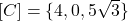.

Step-by-step explanation:

Let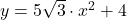, which is a polynomic function, defined as: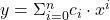(1)

Where: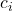– i-th Coefficient.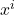– i-th Power.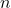– Grade of the polynomial.

We notice that given polynomial has degree 2 and can be rewritten by applying this definition: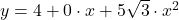Then, the set of coefficients asociated with the given polynomial in ascending order is: